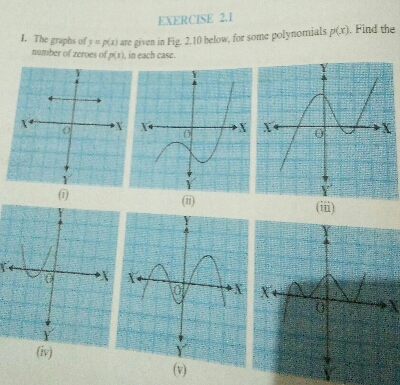# The graphs of $y=p(x)$ are given in Fig. $2.10$ below, for some polynomials $p(x)$. Find the number of zeroes of $p(\bar{x})$, in each case."

Solution:$( i).\ \because$ The given graph does not touch the $x-axis$ at any point. Thus, number of zeroes is $0$.

$( ii).\ \because$ The graph touches the $x-axis$ at one point. Thus, number of zeroes is $1$.

$( iii).\ \because$ The graph touches the $x-axis$ at three points. Thus, the number of zeroes is $3$.

$( iv).\ \because$ The graph touches the $x-axis$ at two points. Thus, the number of zeroes is $2$.

$( v).\ \because$ The graph touches the $x-axis$ at $4$ points. Thus, the number of zeroes is $4$.

$( vi).\ \because$ The graph touches the $x-axis$ at $3$ points. Thus, the number of zeroes is $3$.